CIS 5511. Programming Techniques

Graphs (1)

### 1. Graphs and their representation

A graph consists of vertices (nodes) and edges (links) among them, and represents relations (of the same type) among entities.

Formally, a graph G = (V, E), where V is a set of vertices, and E is a set of edges (from one vertex to another vertex), so |E| ≤ |V|2. The "size" of G is usually represented by both |V| and |E|, with respect to which the computational complexity of each graph algorithm is represented. Sometime f(|V|, |E|) is simplified to f(V, E).

A graph may either be directed or undirected, depending on whether the relation is symmetric or not.

A path is a sequence of end-to-end edges, and its length can be any natural number. A cycle is a path with the same starting and ending vertex, with a length of at least 3 in an undirected graph or 1 in a directed graph.

An undirected graph is connected if there is a path from every vertex to every other vertex. A directed graph is strongly connected if there is a (directed) path from every vertex to every other vertex; it is weakly connected if the graph is connected after all directed edges are turned into undirected edges.

The two common ways to represent a graph is by an adjacency matrix and by an adjacency list. The former uses Θ(|V|2) space and is better for dense graphs, while the latter uses Θ(|V| + |E|) space and is better for sparse graphs.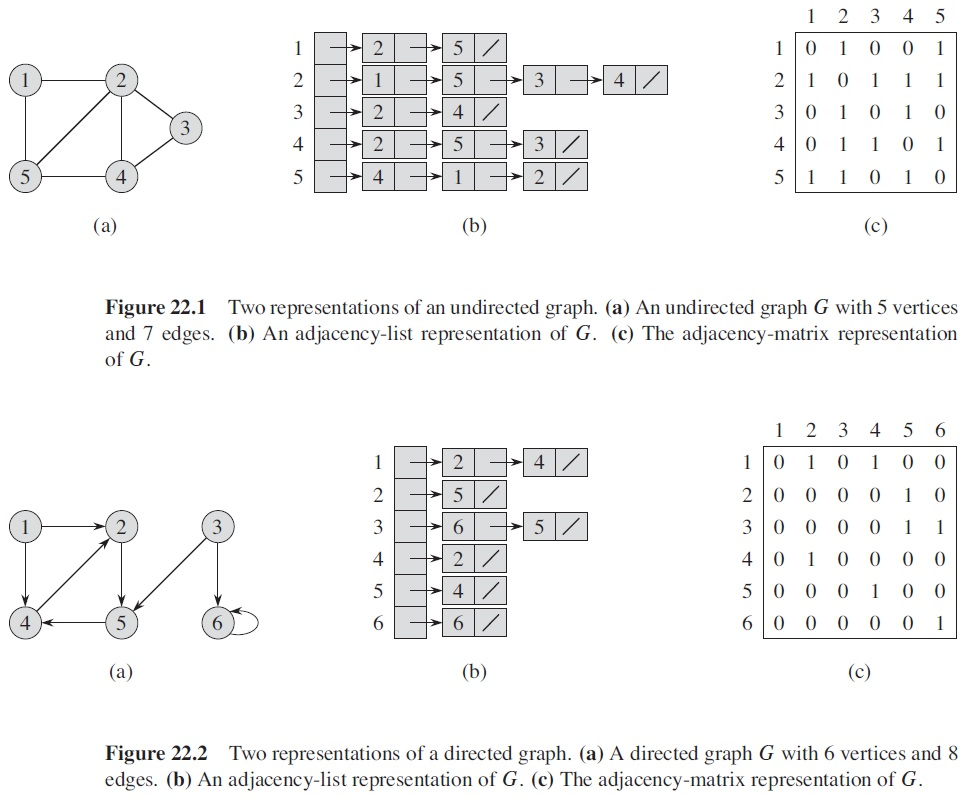A graph is weighted if every edge has a number associated to represent distance, cost, etc., which can be represented by augmenting the adjacency matrix or list.

Graph is more general than tree and linear data structures, though it can be further extended into hypergraph, multigraph, etc.

### 2. Search

In graphs, various search (traversal) algorithms systematically visit every vertex by following the edges.

Breadth-First Search (BFS): starting from a source vertex, visits its neighbors layer-by-layer with increasing distance. A "color" is attached to every node to indicate its status: to be processed (white), being processed (gray), have been processed (black). For each node, its predecessor (π) and distance (d) are recorded (on the path from the source node). A queue is used to hold the nodes under processing.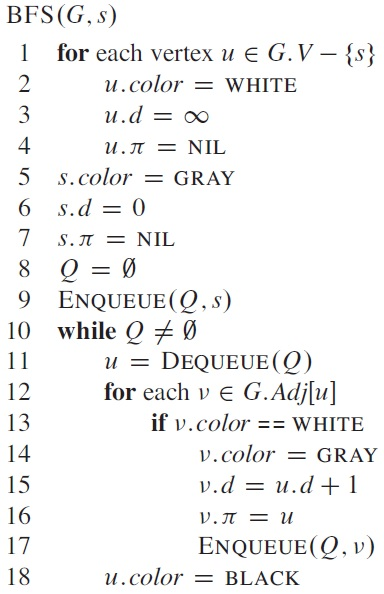Example: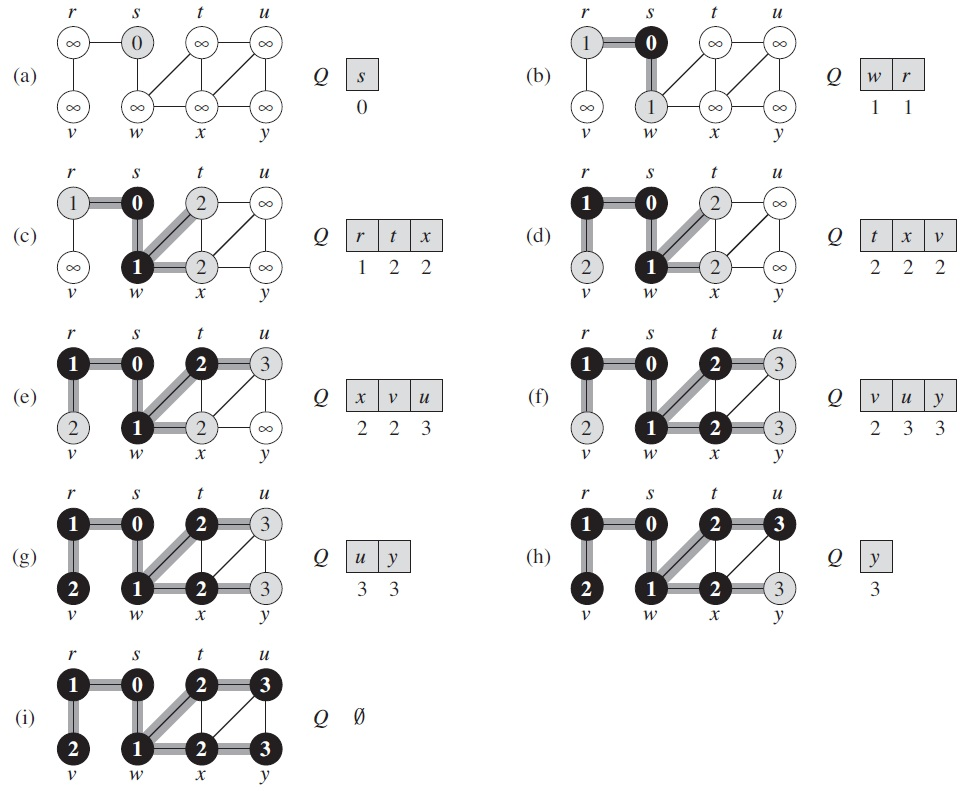Under the assumption that all nodes can be reached from the source node, the running time of BFS is Θ(V + E).

As a by-product, this algorithm also generates a search tree with s as root, and finds the shortest path between s and every reachable vertex in G. In line 13, if color[v] is not WHITE, then a cycle (loop, circle) is detected.

If in search the algorithm tries to go as deep as possible in each step, then it is a Depth-First Search (DFS) algorithm.

The above BFS algorithm can be changed into a DFS algorithm by simply changing the queue into a stack, and each time only processing one successor of the node at the top of the stack.

The following is a recursive DFS, which uses two time-stamps, d and f, to record the time of color changing for each vertex. This information will be used in the other algorithms to be introduced later. This algorithm does not specify a starting node, and may generate a search forest containing more than one tree.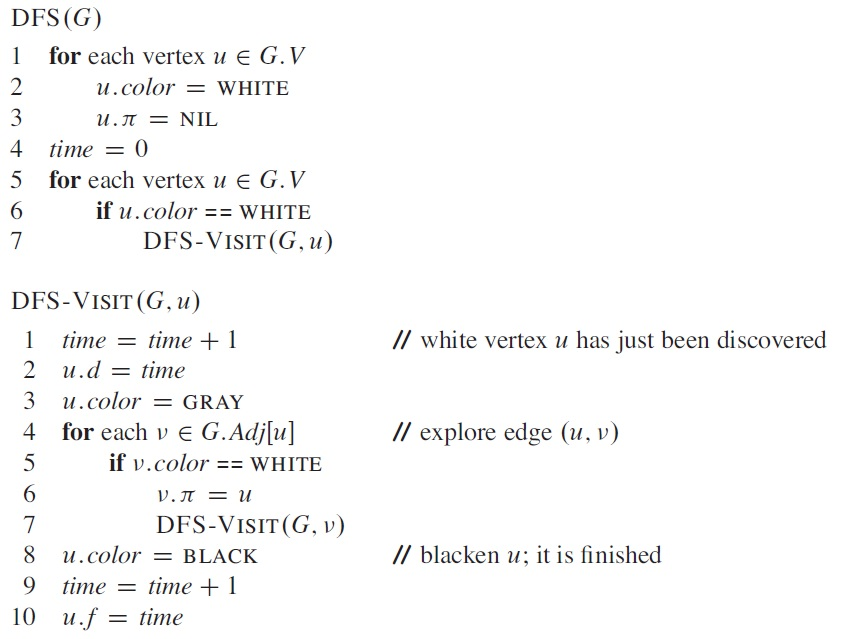Example: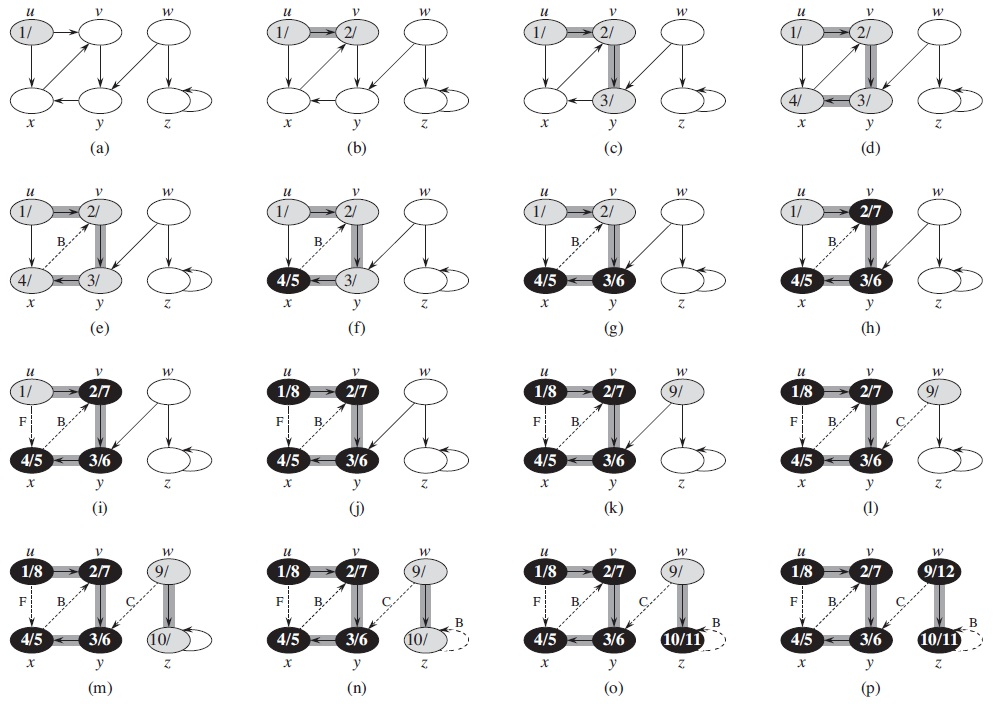The running time of DFS is also Θ(V + E), though this algorithm does not assume the connectivity of the graph.

### 3. Topological sort

A topological sort of a directed acyclic graph ("dag") G = (V, E) is a linear ordering of all its vertices such that if G contains an edge (u, v), then u appears before v in the ordering. If the graph is not acyclic, then no linear ordering is possible.

The following algorithm uses DFS to do topological sorting: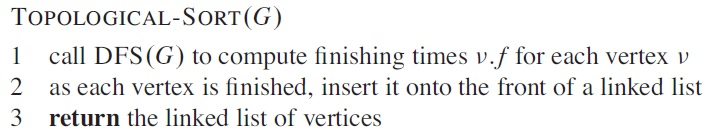Example: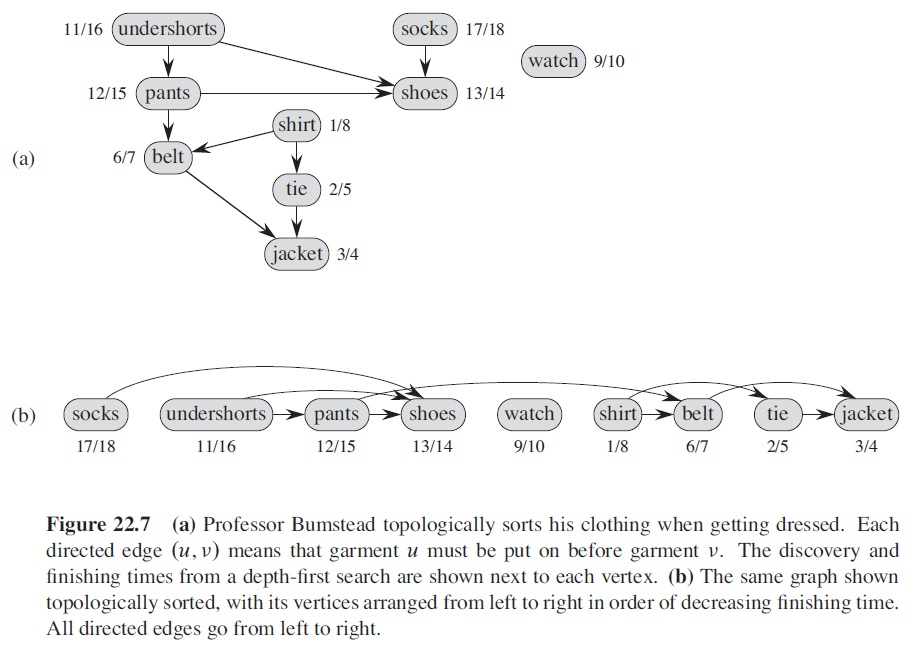The algorithm can be slightly changed, so that the algorithm repeatedly remove vertex v and out-going edges from it, under the condition that there is no in-coming edge to v.

### 4. Strongly connected components

DFS can also be used to decompose a directed graph into its strongly connected components. In the algorithm, the transpose of G, GT, is obtained by reversing the direction of all the edges of G.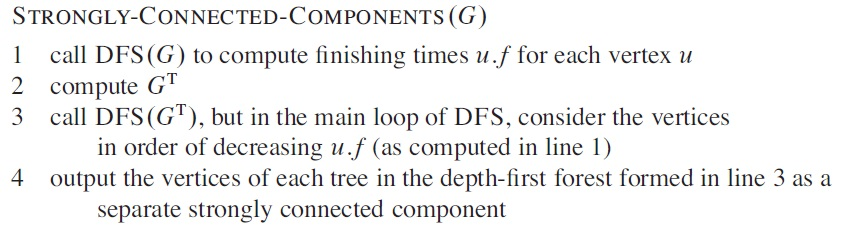Example: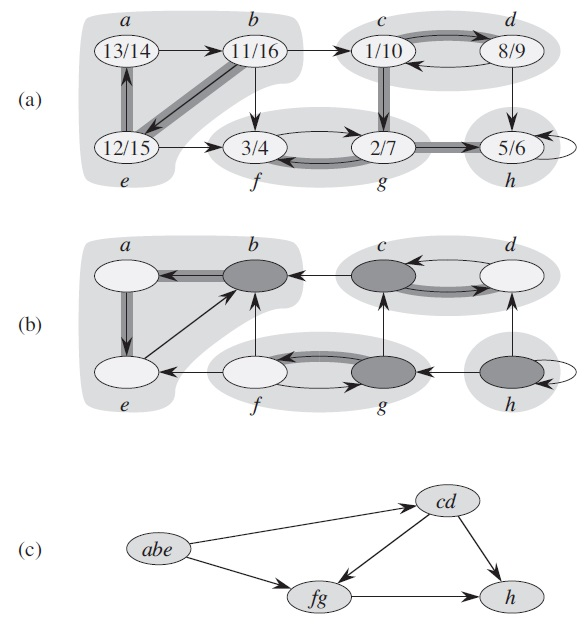### 5. Minimum spanning trees

For an undirected, connected, and weighted graph G = (V, E), its minimum spanning tree is a subgraph G' = (V, T), which is still connected, and with a minimum value of ∑w(u, v) [for (u, v) ∈ T].

Kruskal's algorithm each time adds an edge that have the least w, and connect two previously unconnected subgraphs. The algorithm uses a set to represent a subtree, and Find-Set(u) identifies the set in which u belongs.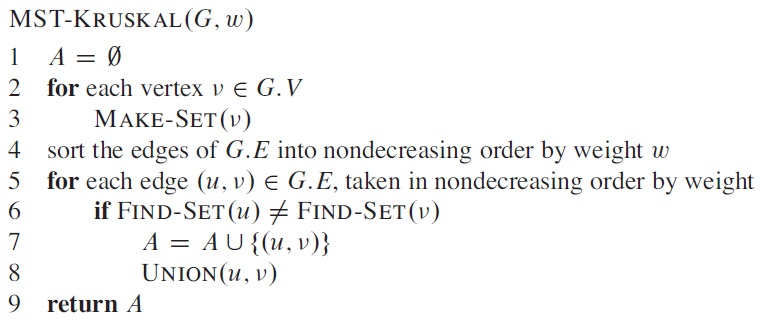Example: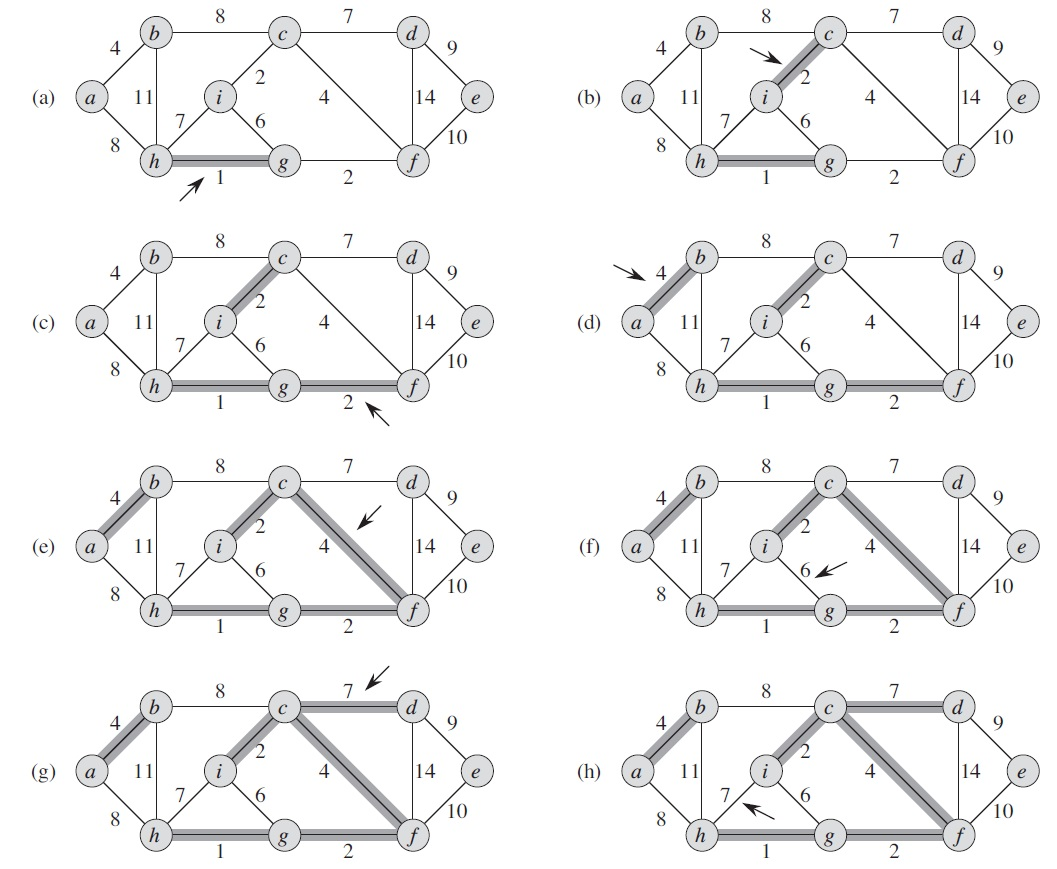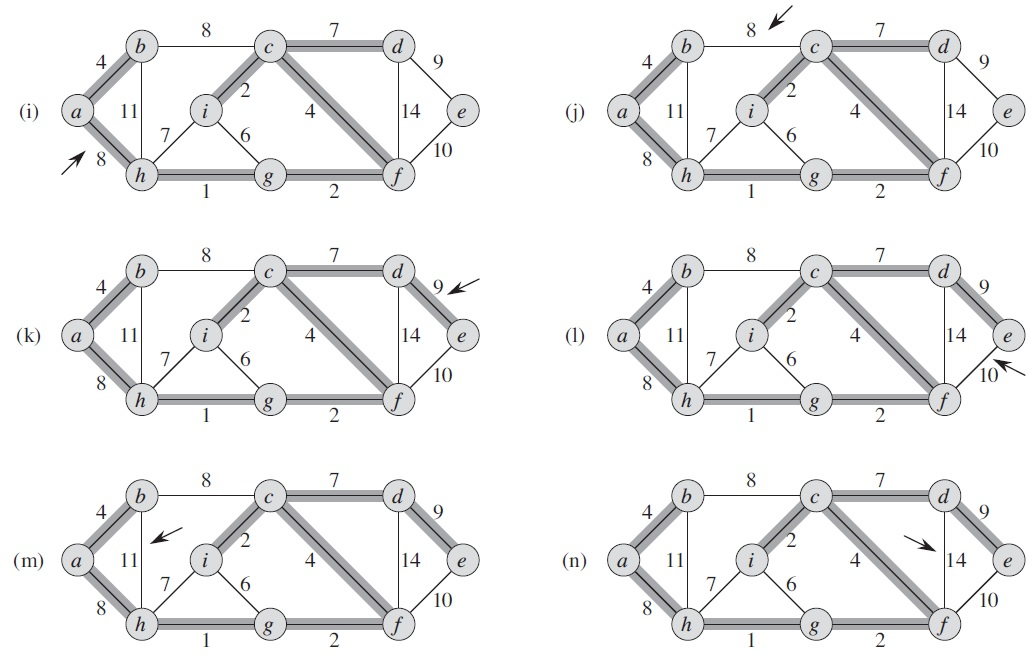Kruskal's algorithm is a greedy algorithm, and takes O(E lg V) time.

Prim's algorithm adds vertices into the tree one by one, starting from a root r.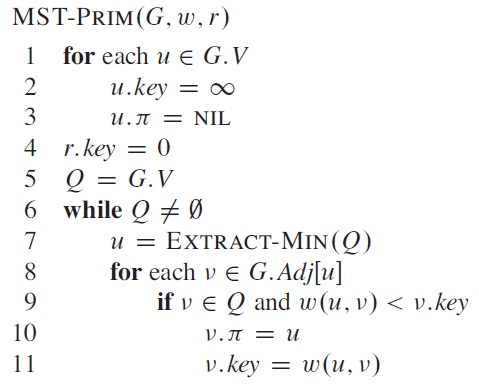For each vertex v, key[v] is the minimum weight of any edge connecting v to a vertex in the tree. In line 7, Extract-Min(Q) takes the vertex with the lowest key value out of Q.

Example: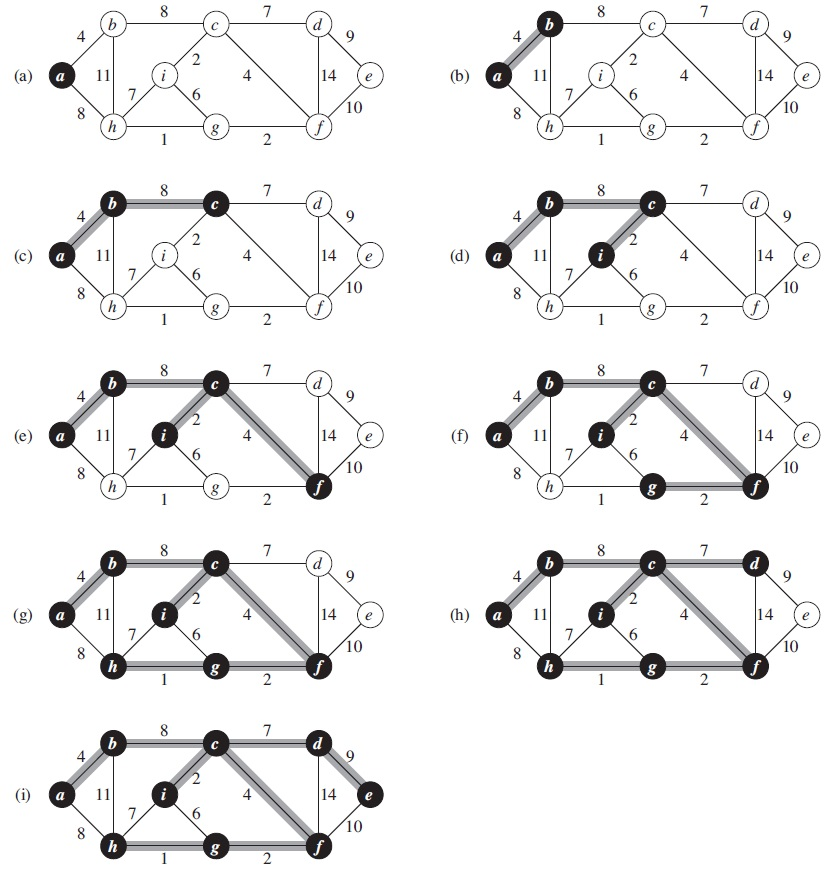Prim's algorithm also takes O(E lg V) time.